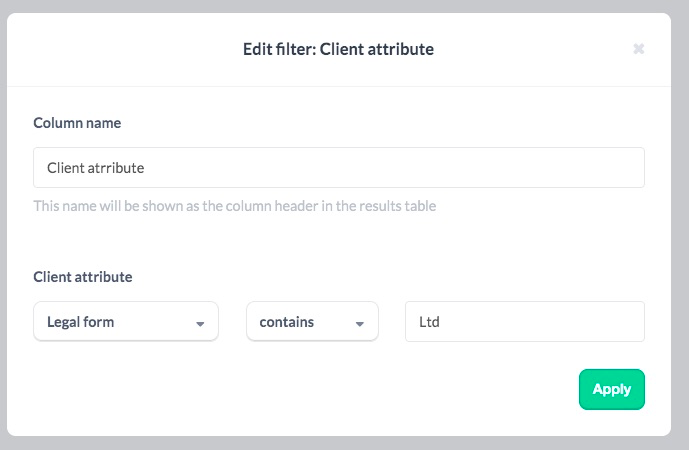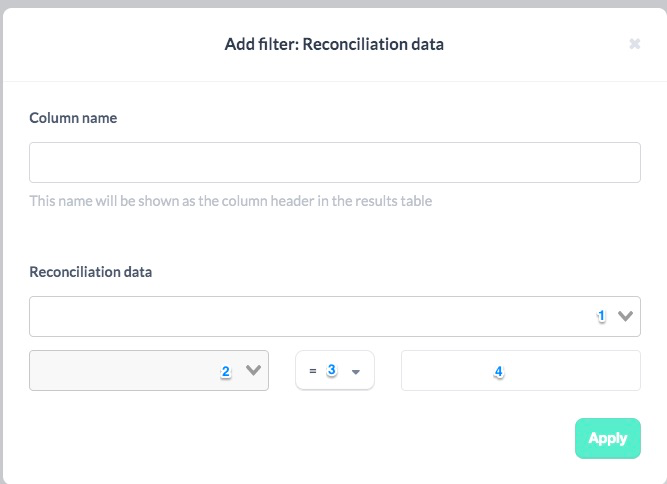Debtor days increasing for 3 months:

Formula:

Column Name: Inc DD (M-2)

Formula: #140000/(-#4+(-period.minus_12p.year_end.accounts.4+#4:12p))*365-#140000:1p/(-#4:1p+(-period.minus_13p.year_end.accounts.4+#4:13p))*365

Comparison: >

Comparison value: 0

Formula:

Column Name: Inc DD (M-1)

Formula: #140000:1p/(-#4:1p+(-period.minus_13p.year_end.accounts.4+#4:13p))*365-#140000:2p/(-#4:2p+(-period.minus_14p.year_end.accounts.4+#4:14p))*365

Comparison: >

Comparison value: 0

Formula:

Column Name: Inc DD

Formula: #140000:2p/(-#4:2p+(-period.minus_14p.year_end.accounts.4+#4:14p))*365-#140000:3p/(-#4:3p+(-period.minus_15p.year_end.accounts.4+#4:15p))*365

Comparison: >

Comparison value: 0

Cash balance declining for 3 months:

Formula:

Column Name: Cash Balance

Formula: #14090

Comparison: Show all

Comparison value: N/A

Formula:

Column Name: Cash Decline (2 months ago)

Formula: #14090:2p-#14090:3p

Comparison: <

Comparison value: 0

Formula:

Column Name: Cash Decline (last month)

Formula: #14090:1p-#14090:2p

Comparison: <

Comparison value: 0

Formula:

Column Name: Cash Decline (this month)

Formula: #14090-#14090:1p

Comparison: <

Comparison value: 0

Loss making companies:

Formula:

Column Name: Loss value

Formula: #4__9

Comparison: >

Comparison value: 0

Directors current account- P11D:

Formula:

Column Name: Directors Loan value

Formula: #140301__140302

Comparison: >

Comparison value: 10000

Client attribute:

Column Name: Client attribute

Client attribute: Legal form

Box 2: contains

Box 3: LtdNote: The following relies on the reporting framework and number of employees having been included as a question in the Insights/Core Data template

Audit threshold:Reconciliation data:

Step 1: Select the relevant reconciliation from the dropdown (according to what it is called in your environment)

Step 2: Select the 'framework' dropdown

Step 3: es exactly

Step 4: FRS 102 1A

Reconciliation data:

Step 1: Select the relevant reconciliation from the dropdown (according to what it is called in your environment)

Step 2: Select the 'Number of employees' dropdown

Step 3: >

Step 4: 50

Formula:

Column Name: Financial metric

Formula:MAX(#1,5100000) + MAX(-#4,10200000)

Comparison: >

Comparison value: 15300000

Formula:

Column Name: Total assets

Formula: #1

Comparison: >

Comparison value: 0

Formula:

Column Name: Revenue

Formula: -#4

Comparison: >

Comparison value: 0